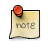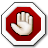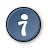Navigation C API Pages Python bindings Applications

## Python GFX module

The python binding maps mostly to the C API with the GP_ prefix stripped.

The gfx module adds methods to the gfx pixmap submodule.You may want to see the coordinate system first.

### Drawing functions

All drawing functions takes a pixel value (to describe color) which can be obtained, for a particular pixel type (pixmap), from a RGB triplet.

All drawing functions are clipped. Drawing outside of a pixmap is no-op.Drawing functions takes strictly integer coordinates, make sure that all divisions are integer divisions (i.e. use // instead of /) to ensure portability with Python 3.X.

#### Line Based Primitives

```import gfxprim.gfx as gfx

pixmap.gfx.HLine(x0, x1, y, pixel)
```

Draws a horizontal line from x0 to x1 at y.

```import gfxprim.gfx as gfx

pixmap.gfx.VLine(x, y0, y1, pixel)
```

Draws a vertical line from y0 to y1 at x.

```import gfxprim.gfx as gfx

pixmap.gfx.Line(x0, y0, x1, y1, pixel)
```

Draws a line from x0, y0 to x1, y1.

```import gfxprim.gfx as gfx

pixmap.gfx.Rect(x0, y0, x1, y1, pixel)
```

Draws a rectangle defined by two points.

```import gfxprim.gfx as gfx

pixmap.gfx.Triangle(x0, y0, x1, y1, x1, y2, pixel)
```

Draws a triangle defined by three points.

```import gfxprim.gfx as gfx

pixmap.gfx.Tetragon(x0, y0, x1, y1, x1, y2, x3, y3, pixel)
```

Draws a tetragon defined by four points.

```import gfxprim.gfx as gfx

pixmap.gfx.Polygon([x0, y0, x1, y1, ...], pixel)

pixmap.gfx.Polygon([(x0, y0), (x1, y1), ...], pixel)
```

Draws a polygon defined by points, points can be either flat list of 2N integers or list of N tuples.

```import gfxprim.gfx as gfx

pixmap.gfx.Circle(x, y, r, pixel)
```

Draws a circle.

```import gfxprim.gfx as gfx

pixmap.gfx.Ellipse(x, y, a, b, pixel)
```

Draws an ellipse.

```import gfxprim.gfx as gfx

pixmap.gfx.Ring(x, y, r1, r2, pixel)
```

Draws a ring.

#### Filled Primitives

```import gfxprim.gfx as gfx

pixmap.gfx.Fill(pixel)
```

Fills pixmap with particular pixel value.

```import gfxprim.gfx as gfx

pixmap.gfx.FillRect(x0, y0, x1, y1, pixel)
```

Fills a rectangle defined by two points.

```import gfxprim.gfx as gfx

pixmap.gfx.FillTriangle(x0, y0, x1, y1, x1, y2, pixel)
```

Draws a filled triangle defined by three points.

```import gfxprim.gfx as gfx

pixmap.gfx.FillTetragon(x0, y0, x1, y1, x1, y2, x3, y3, pixel)
```

Draws a filled tetragon defined by four points.

```import gfxprim.gfx as gfx

pixmap.gfx.FillPolygon([x0, y0, x1, y1, ...], pixel)

pixmap.gfx.FillPolygon([(x0, y0), (x1, y1), ...], pixel)
```

Fills a polygon defined by points, points can be either flat list of 2N integers or list of N tuples.

```import gfxprim.gfx as gfx

pixmap.gfx.FillCircle(x, y, r, pixel)
```

Fills a circle.

```import gfxprim.gfx as gfx

pixmap.gfx.FillEllipse(x, y, a, b, pixel)
```

Fills an ellipse.

```import gfxprim.gfx as gfx

pixmap.gfx.FillRing(x, y, r1, r2, pixel)
```

Fills a ring, i.e. area enclosed between two circles.See gfx module example.